### ZEROMODEL Statement

ZEROMODEL dependent variable<zero-inflated regressors> </options> ;

The ZEROMODEL statement is required if either ZIP or ZINB is specified in the DIST= option in the MODEL statement. If ZIP or ZINB is specified, then the ZEROMODEL statement must follow immediately after the MODEL statement. The dependent variable in the ZEROMODEL statement must be the same as the dependent variable in the MODEL statement.

The zero-inflated (ZI) regressors appear in the equation that determines the probability (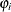) of a zero count. Each of these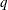variables has a parameter to be estimated in the regression. For example, let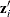be theth observation’s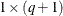vector of values of theZI explanatory variables (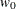is set to 1 for the intercept term). Thenis a function of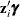, where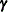is the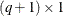vector of parameters to be estimated. (The ZI intercept is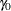; the coefficients for theZI covariates are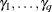.) If this option is omitted, then only the intercept termis estimated. The “Parameter Estimates” table in the displayed output gives the estimates for the ZI intercept and ZI explanatory variables; they are labeled with the prefix Inf_. For example, the ZI intercept is labeled Inf_intercept. If you specify Age (a variable in your data set) as a ZI explanatory variable, then the “Parameter Estimates” table labels the corresponding parameter estimate Inf_Age.

The following options can be specified in the ZEROMODEL statement following a slash (/):

specifies the distribution function used to compute probability of zeros. The following distribution functions are supported:

LOGISTIC

specifies the logistic distribution.

NORMAL

specifies the standard normal distribution.

If this option is omitted, then the default ZI link function is logistic.

OFFSET=variable

specifies a variable in the input data set to be used as a zero-inflated (ZI) offset variable. The ZI offset variable is included as a term, with coefficient restricted to 1, in the equation that determines the probability () of a zero count. The ZI offset variable cannot be the response variable, the offset variable (if any), or one of the explanatory variables. The name of the data set variable used as the ZI offset variable is displayed in the “Model Fit Summary” output, where it is labeled as Inf_offset.